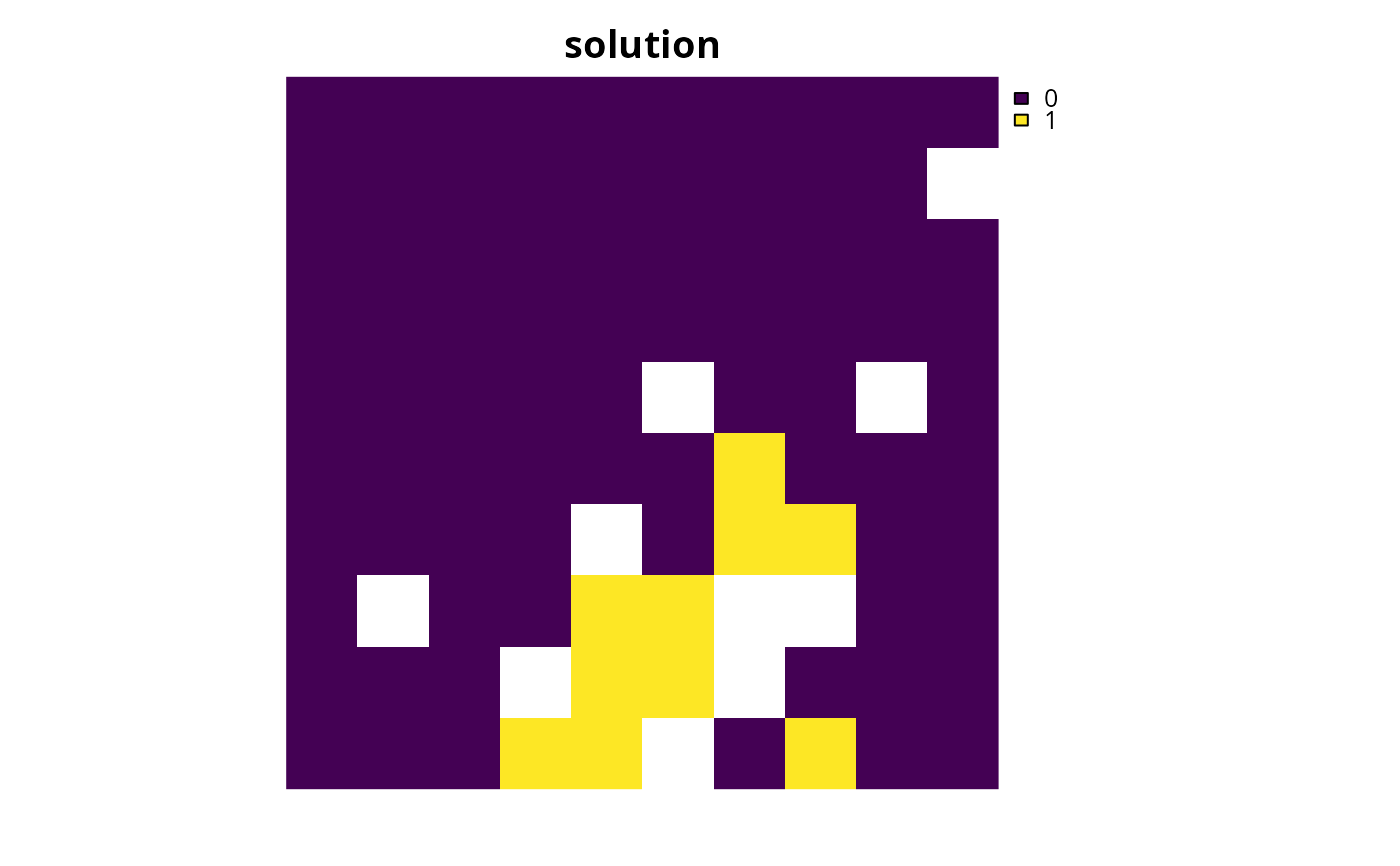Specify that the SYMPHONY software (Ralphs & Güzelsoy 2005) -- using the Rsymphony package -- should be used to solve a conservation planning problem(). This function can also be used to customize the behavior of the solver. It requires the Rsymphony package to be installed.

add_rsymphony_solver(
x,
gap = 0.1,
time_limit = .Machine$integer.max, first_feasible = FALSE, verbose = TRUE ) ## Arguments x problem() (i.e., ConservationProblem) object. gap numeric gap to optimality. This gap is relative and expresses the acceptable deviance from the optimal objective. For example, a value of 0.01 will result in the solver stopping when it has found a solution within 1% of optimality. Additionally, a value of 0 will result in the solver stopping when it has found an optimal solution. The default value is 0.1 (i.e., 10% from optimality). time_limit numeric time limit (seconds) for generating solutions. The solver will return the current best solution when this time limit is exceeded. The default value is the largest integer value (i.e., .Machine$integer.max), effectively meaning that solver will keep running until a solution within the optimality gap is found.

first_feasible

logical should the first feasible solution be be returned? If first_feasible is set to TRUE, the solver will return the first solution it encounters that meets all the constraints, regardless of solution quality. Note that the first feasible solution is not an arbitrary solution, rather it is derived from the relaxed solution, and is therefore often reasonably close to optimality. Defaults to FALSE.

verbose

logical should information be printed while solving optimization problems? Defaults to TRUE.

## Value

Object (i.e., ConservationProblem) with the solver added to it.

## Details

SYMPHONY is an open-source mixed integer programming solver that is part of the Computational Infrastructure for Operations Research (COIN-OR) project. The Rsymphony package provides an interface to COIN-OR and -- unlike dependencies for other solvers -- is available on CRAN. For information on the performance of different solvers, please see Schuster et al. (2020) for benchmarks comparing the run time and solution quality of different solvers when applied to different sized datasets.

See solvers for an overview of all functions for adding a solver.

Other solvers: add_cbc_solver(), add_cplex_solver(), add_default_solver(), add_gurobi_solver(), add_lsymphony_solver

## Examples

# \dontrun{
data(sim_pu_raster, sim_features)

# create problem
p <- problem(sim_pu_raster, sim_features) %>%